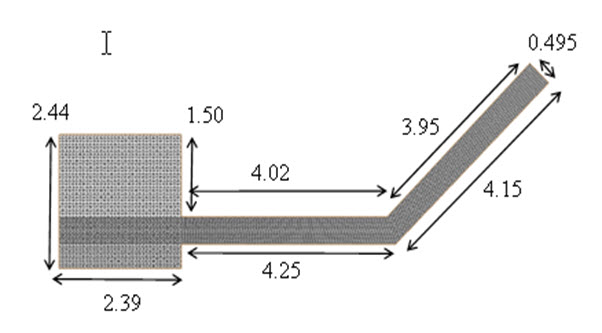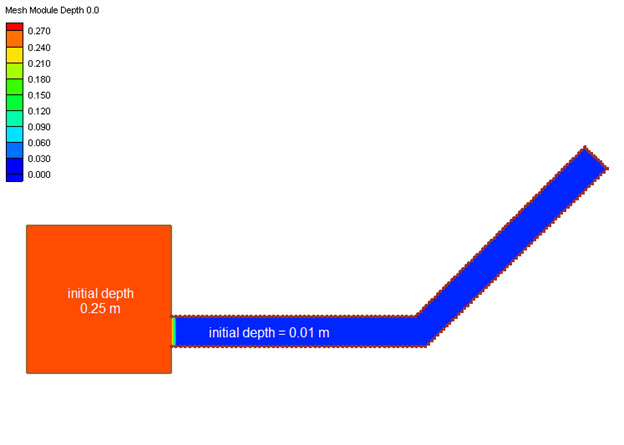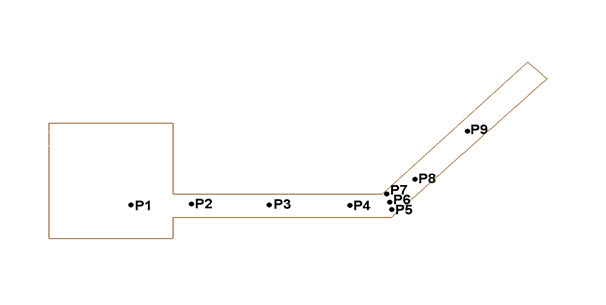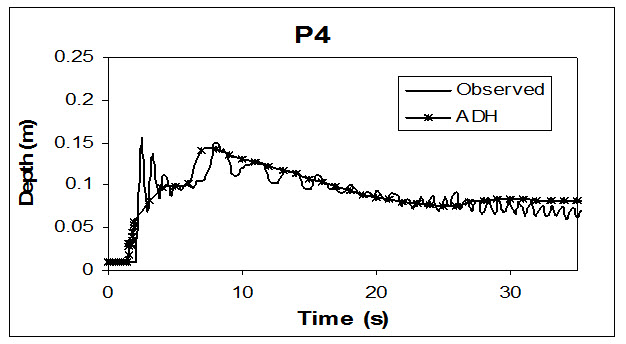## Dam Failure over Wet Bed with Angled Flume

Reference

• Brufau P. and Garcia-Navarro P. (2000). “Two-dimensional Dam Break Flow Simulation.” International Journal for Numerical Methods in Fluids, 33, 35-57.

Domain

• Square reservoir and a 45 deg bend channel
• Reservoir length = 2.44 and width = 2.39 m
• Straight channel length = 4.25 m and width = 0.495 m
• Angled channel length = 4.15 m and width = 0.495 mInitial Conditions

• Initial reservoir water depth = 0.25 m
• Initial channel water depth = 0.01 m
• Friction defined by Manning's
• flume bottom n = 0.0095
• flume side wall n = 0.0195Boundary Conditions

• Reservoir release at t = 0 s

Results

• Point water surface elevation comparisons to measured data over time